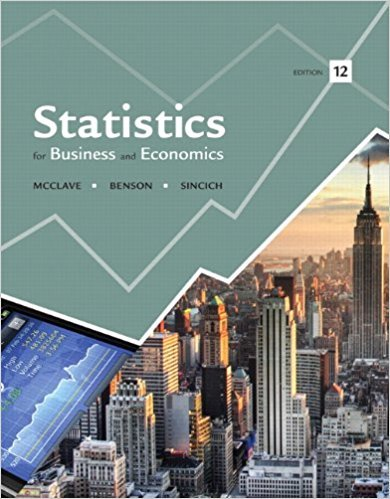×
×

# Refer to Exercise 5.3.a. Find the sampling distribution ofISBN: 9780321826237 51

## Solution for problem 13E Chapter 5

Statistics for Business and Economics | 12th Edition

• Textbook Solutions
• 2901 Step-by-step solutions solved by professors and subject experts
• Get 24/7 help from StudySoup virtual teaching assistantsStatistics for Business and Economics | 12th Edition

4 5 1 290 Reviews
26
1
Problem 13E

Refer to Exercise 5.3.

a. Find the sampling distribution of s2.

b. Find the population variance σ2.

c. Show that s2 is an unbiased estimator of σ2.

d. Find the sampling distribution of the sample standard deviation s.

e. Show that s is a biased estimator of σ.Step-by-Step Solution:

Step 1 of 5</p>

a) We have to find the sampling distribution of s2

From the data given in exercise we have find s2Now calculate the s2 values for each sample

For the sample (1,1)Then s2 =[(1-1)2+(1-1)2]/1

=0

For the sample (1,2)Then s2 =[(1-15)2+(2-1.5)2]/1

=0.5

Calculate the  s2 values for all the remaining samples

 s2 p(s2) s2 p(s2) 0 0.04 0.5 0.04 0.5 0.06 2 0.02 2 0.04 4.5 0.04 4.5 0.04 2 0.06 8 0.02 0.5 0.04 0.5 0.06 0 0.04 0 0.09 0.5 0.02 0.5 0.06 8 0.02 2 0.06 4.5 0.03 4.5 0.03 2 0.02 2 0.04 0.5 0.02 0.5 0.06 0 0.01 0 0.04

Now the sampling distribution of s2  is

 s2 0 0.5 2 4.5 8 p(s2) 0.22 0.36 0.24 0.14 0.04

Step 2 of 5</p>

b) We have to find population varianceNow==1.61

Step 3 of 3

##### ISBN: 9780321826237

This textbook survival guide was created for the textbook: Statistics for Business and Economics , edition: 12. Statistics for Business and Economics was written by and is associated to the ISBN: 9780321826237. The answer to “Refer to Exercise 5.3.a. Find the sampling distribution of s2.b. Find the population variance ?2.c. Show that s2 is an unbiased estimator of ?2.d. Find the sampling distribution of the sample standard deviation s.e. Show that s is a biased estimator of ?.” is broken down into a number of easy to follow steps, and 43 words. This full solution covers the following key subjects: Find, distribution, Sampling, estimator, refer. This expansive textbook survival guide covers 15 chapters, and 1630 solutions. The full step-by-step solution to problem: 13E from chapter: 5 was answered by , our top Business solution expert on 07/21/17, 05:42AM. Since the solution to 13E from 5 chapter was answered, more than 313 students have viewed the full step-by-step answer.

Unlock Textbook Solution